# 「牛客网」45道JS能力测评经典题总结

## 前言我也把常用的数组方法和字符串方法贴在这里，可以自测掌握程度

## 1、查找数组元素位置

``````// 方法一
function indexOf(arr, item) {
if(Array.prototype.indexOf){// 判断浏览器是否支持indexOf方法
return arr.indexOf(item);
}else{
for(var i=0;i<arr.length;i++){
if(arr[i]===item){
return i;
}
}
}
return -1;
}
// 方法二
function indexOf(arr, item) {
if(Array.prototype.indexOf){// 判断浏览器是否支持indexOf方法
return arr.indexOf(item);
} else if (arr.indexOf(item) > 0) {
return arr.indexOf(item)
}else{
return -1
}
}

`indexOf()`方法返回在数组中可以找到一个给定元素的第一个索引，如果不存在，则返回-1。

`````` arr.indexOf(searchElement) //查找searchElement元素在数组中的第一个位置
arr.indexOf(searchElement[, fromIndex = 0]) //从fromIndex开始查找searchElement元素在数组中的第一个位置

``````str.indexOf(searchValue[, fromIndex])

• searchValue：一个字符串表示被查找的值。
• fromIndex （可选）：表示调用该方法的字符串中开始查找的位置。可以是任意整数。默认值为 0。如果 fromIndex < 0 则查找整个字符串（如同传进了 0）。如果 fromIndex >= str.length，则该方法返回 -1，除非被查找的字符串是一个空字符串，此时返回 str.length。

## 2、添加元素(末尾添加)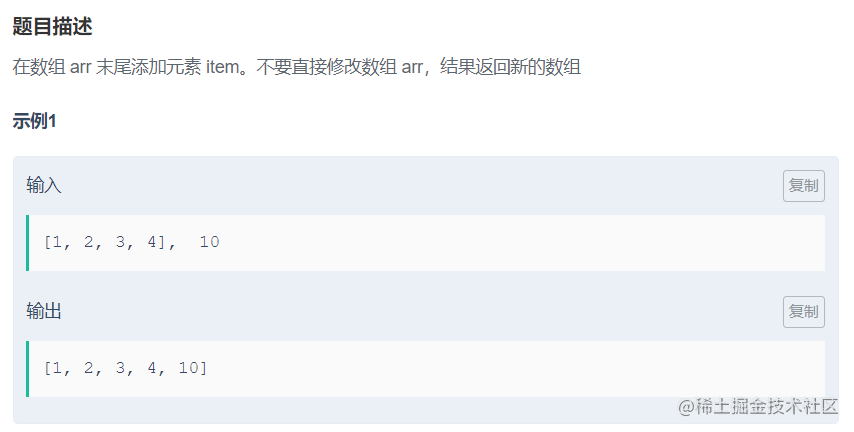方法一：普通的for循环拷贝+push

``````function append(arr, item) {
let resArr = []
for(let i = 0;i<arr.length;i++){
resArr.push(arr[i])
}
resArr.push(item)
return resArr
}

``````function append(arr, item) {
return arr.concat(item);
}

``````function append(arr, item) {
let newArr = arr.slice(0);  // slice(start, end)浅拷贝数组
newArr.push(item);
return newArr;
}

``````function append(arr, item) {
let resArr = [...arr,item]
return resArr
}

## 3、移除数组中的元素(返回原数组)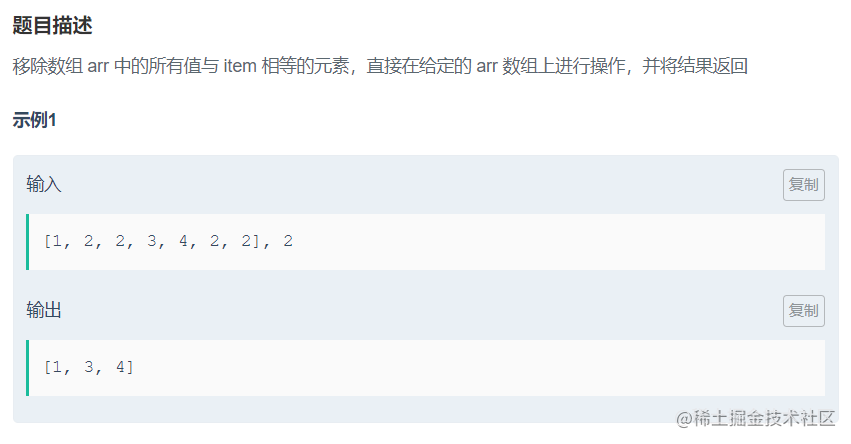这里需要注意理解题目，是直接操作原数组，所以不能出现newArr

``````function removeWithoutCopy(arr, item) {
for(let i=arr.length;i>=0;i--){
if(arr[i]==item){
arr.splice(i,1);
}
}
return arr;
}

``````function removeWithoutCopy(arr, item) {
for(let i = 0; i< arr.length; i++) {
if(arr[i]===item) {
arr.splice(i,1);
i--;
}
}
return arr;
}

## 4、移除数组中的元素(返回新的数组)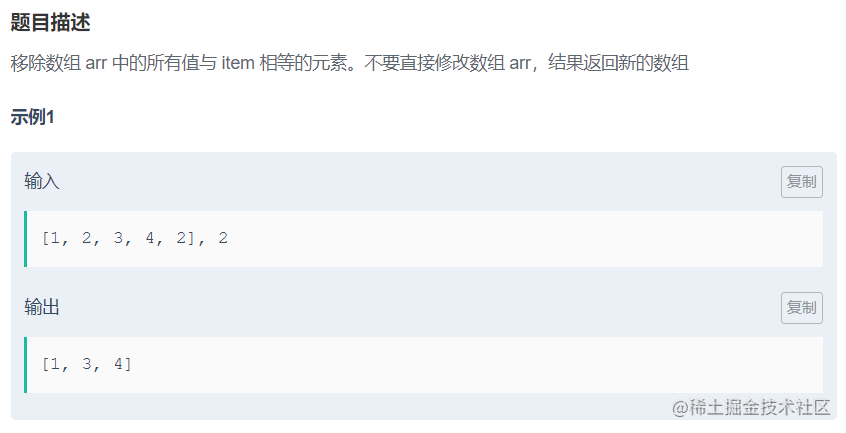方法一：filter过滤

``````function remove(arr, item) {
return arr.filter(res =>{
return res != item;
})
}

``````function remove(arr, item) {
let resArr = []
for(let i = 0;i<arr.length;i++){
if(arr[i]!== item){
resArr.push(arr[i])
}
}
return resArr
}

``````function remove(arr, item) {
let resArr=[];
arr.forEach(v=>{
if(v!==item){
resArr.push(v);
}
})
return resArr;
}

``````function remove(arr,item){
let resArr= arr.slice(0);
for(let i=0;i<resArr.length;i++){
if(resArr[i] == item){
resArr.splice(i,1);
i--;
}
}
return resArr;
}

## 5、数组求和

``````function sum(arr) {
let res = 0
for(let i=0;i<=arr.length;i++){
res +=arr[i]
}
return res
}

``````function sum(arr) {
let res = 0
arr.forEach((value,index,array)=>{
array[index] == value;    //结果为true
res+=value;
});
return res;
};

reduce() 方法接收一个函数作为累加器，数组中的每个值（从左到右）开始缩减，最终计算为一个值，具体可以看看es6相关知识。

``````function sum(arr) {
return arr.reduce((pre,cur)=>{
return pre+cur;
})
}

eval() 函数可计算某个字符串，并执行其中的的 JavaScript 代码。

``````function sum(arr) {
return eval(arr.join("+"));
}

## 6、删除数组最后一个元素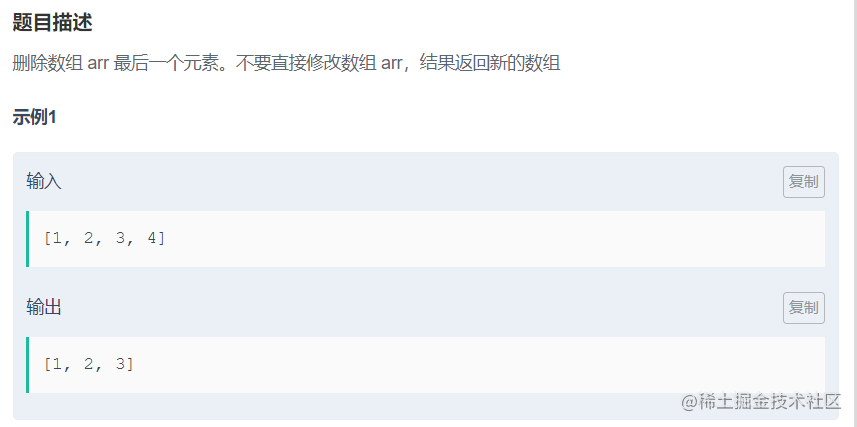方法一：slice

``````function truncate(arr) {
return arr.slice(0,arr.length-1)
}

``````function truncate(arr) {
let resArr = arr.concat()
// let resArr = arr.slice(0)
resArr.pop()
return resArr
}

## 7、添加元素(开头添加)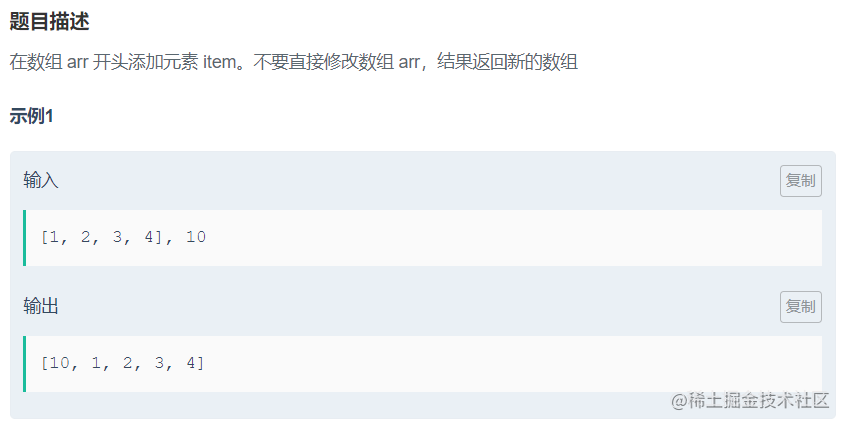concat/slice/arr.join().split(',')+unshift

``````  function prepend(arr, item) {
// let resArr = arr.slice(0);
// let resArr = arr.concat()
let resArr = arr.join().split(',')
resArr.unshift(item);
return resArr;
}

## 8、删除数组第一个元素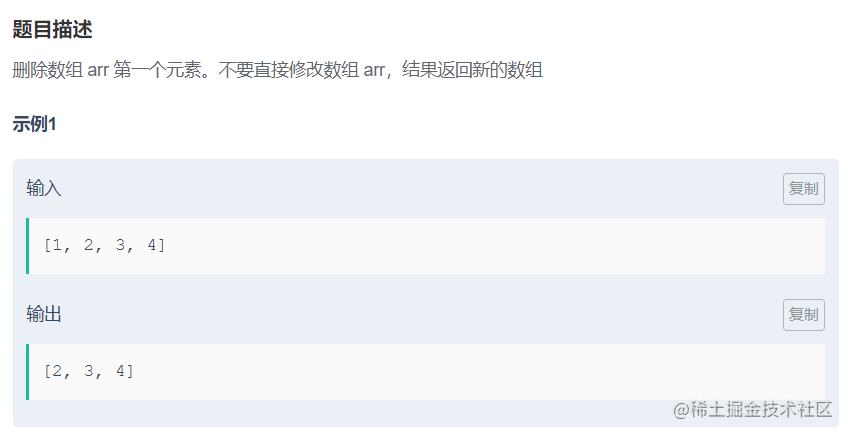与第7题大同小异，玩不出太多花样，如你有其他好玩的方法，欢迎留言

``````function curtail(arr) {
let resArr = arr.slice(0)
resArr.shift()
return resArr
}

## 9、合并数组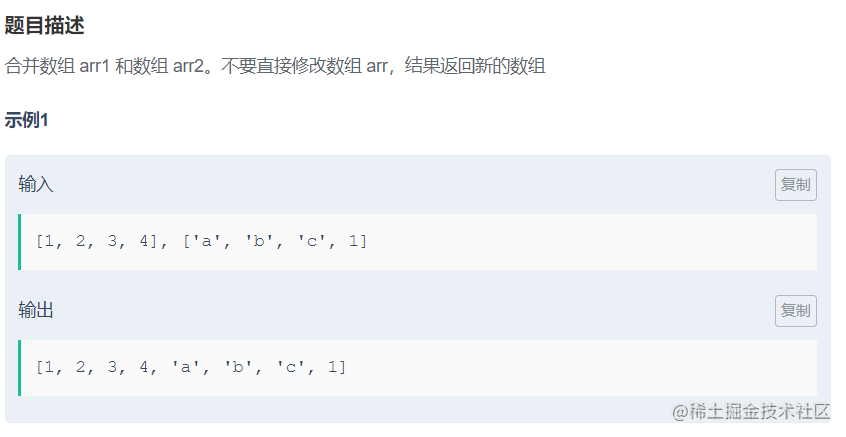方法很多，欢迎大佬们留言评论

``````function concat(arr1, arr2) {
let resArr = arr1.concat(arr2)
return resArr
}

``````function concat(arr1, arr2) {
let resArr = [...arr1,...arr2]
return resArr
}

``````function concat(arr1, arr2) {
let resArr = arr1.slice(0);
[].push.apply(resArr,arr2);
return resArr;
}

## 10、添加元素(指定位置添加)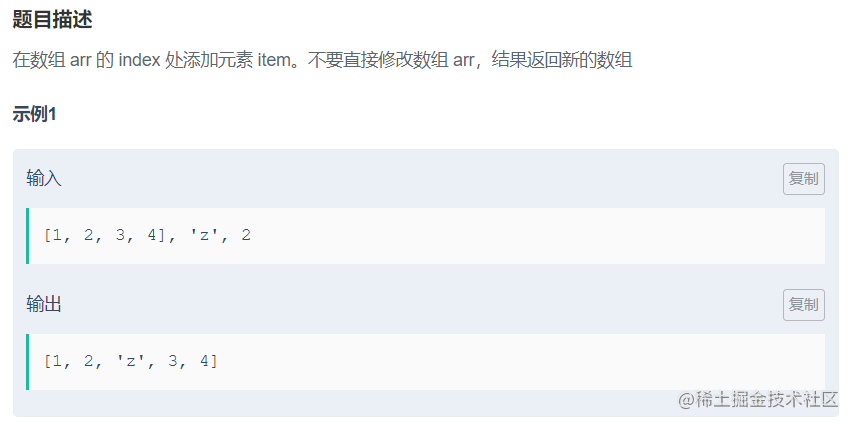方法一：先复制前0~index个元素，将item元素插入之后，再拼接index之后的元素

``````function insert(arr, item, index) {
let resArr = arr.slice(0,index)
resArr.push(item)
resArr = resArr.concat(arr.slice(index))
return resArr
}

``````function insert(arr, item, index) {
let  resArr = arr.slice(0);//
resArr.splice(index,0,item);
return resArr;
}

``````function insert(arr, item, index) {
let resArr=[];
[].push.apply(resArr, arr);
resArr.splice(index,0,item);
return resArr;
}

## 11、计数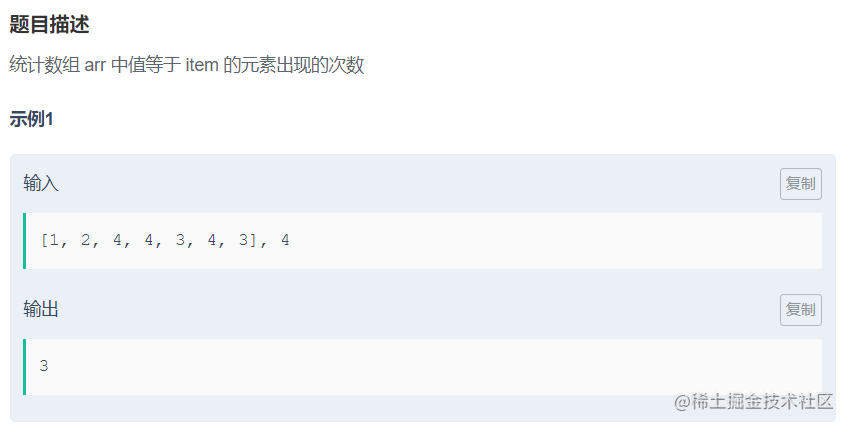方法一：普通for循环

``````function count(arr, item) {
let reSCount = 0
for(let i = 0;i<=arr.length;i++){
if(arr[i] === item){
reSCount++
}
}
return reSCount
}

``````function count(arr, item) {
let resCount=0;
arr.forEach(v => {
if(v==item){
resCount++;
}
});
return resCount;
}

``````function count(arr, item) {
let res = arr.filter(v => {
return v === item
});
return res.length;
}

``````function count(arr, item) {
let resCount = 0
arr.map(v => {
if(v === item){
resCount++
}
});
return resCount;
}

map函数和filter有点像，但是map是对数组中的所有元素进行复核函数条件的处理，最终得到的是一个新数组，元素个数不变。

filter函数虽然也是返回一个新数组，但是元素的个数等于复核函数条件的元素总和。

`````` function count(arr, item) {
let res = arr.reduce( (init,curr)=> {
//如果当前置等于item，该函数值加一
return curr === item ? init+1:init;
},0)
return res;
}

## 12、查找重复元素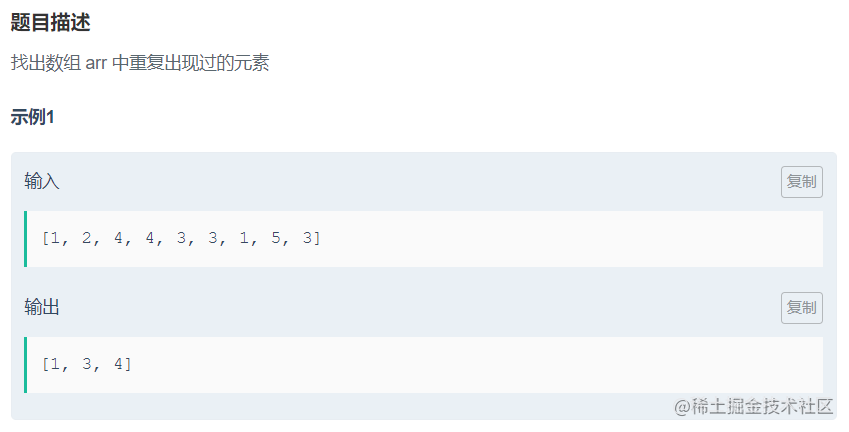方法一：for/for in/+sort 先进行排序，然后判断排序之后的前一个数据是否等于后一个数据，如果是且结果数组没有这个元素

``````//for 运行时间：1596ms 占用内存：77772k
function duplicates(arr) {
let resArr = [];
arr.sort();
for(let i=0;i<arr.length;i++){
if(arr[i]==arr[i-1] && resArr.indexOf(arr[i])==-1){
resArr.push(arr[i]);
}
}
return resArr;
}
//for in  运行时间：1132ms占用内存：77868k
function duplicates(arr) {
let resArr = [];
arr.sort();
for(i in arr){
if(arr[i]==arr[i-1] && resArr.indexOf(arr[i])==-1){
resArr.push(arr[i]);
}
}
return resArr;
}

``````// 运行时间：1184ms 占用内存：77772k
function duplicates(arr) {
var resArr=[];
arr.forEach(v => {
//判断原数组是否有重复数据
//判断结果数组是否已经具有该数据
if(arr.indexOf(v) !=arr.lastIndexOf(v) && resArr.indexOf(v) == -1){
resArr.push(v);
}
});
return resArr;
}

``````// 运行时间：1129ms 占用内存：77776k
function duplicates(arr) {
let b = [];
let resArr = [];
for (let i= 0; i<arr.length; i++){
b[i] = arr.reduce( (init,curr)=> {
//如果当前置等于item，该函数值加一
return curr === arr[i] ? init+1:init;
},0)
if (b[i] > 1 && resArr.indexOf(arr[i]) === -1){
resArr.push(arr[i]);
}
}
return resArr;
}

## 13、求二次方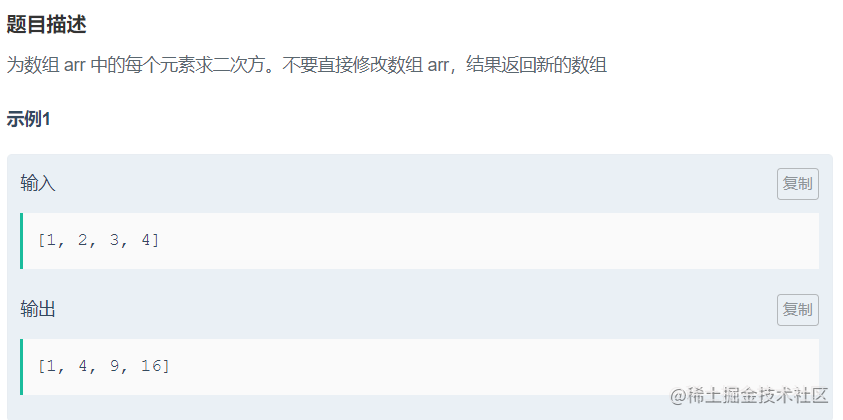方法一：for/forEach

``````// 运行时间：1466ms 占用内存：77772k
function square(arr) {
var res = [];
for(var i in arr){
res.push(arr[i]*arr[i]);
}
return res;
}
// forEach 运行时间：1130ms 占用内存：77772k
function square(arr) {
var resArr = [];
arr.forEach((e)=>{
resArr.push(e*e);
});
return resArr;
}

``````// 运行时间：1433ms 占用内存：78004k
function square(arr) {
//let resArr = arr.slice(0);
let resArr = arr.map((e,index,array)=>{
return e*e;
});
return resArr;
}

## 14、查找元素位置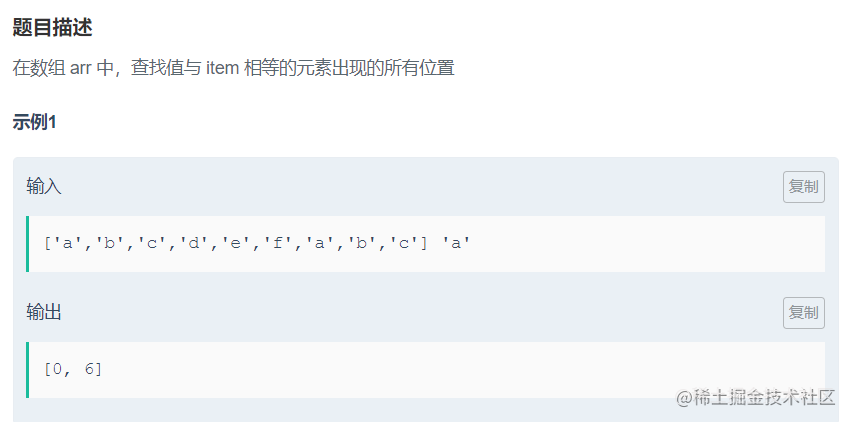方法一:for

``````//运行时间：1139ms 占用内存：77772k
function findAllOccurrences(arr, target) {
let resArr = [];
for(let i=0;i < arr.length;i++){
if(arr[i] === target){
resArr.push(i);
}
}
return resArr;
}

``````// 运行时间：1135ms 占用内存：77776k
function findAllOccurrences(arr, target) {
let resArr = [];
arr.forEach((v,index)=>{
if(v === target){
resArr.push(index)
}
})
return resArr;
}

## 15、避免全局变量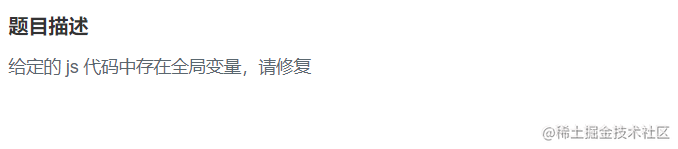原代码：

``````function globals() {
myObject = {
name : 'Jory'
};

return myObject;
}

``````function globals() {
let  myObject = {
name : 'Jory'
};

return myObject;
}

## 16、正确的函数定义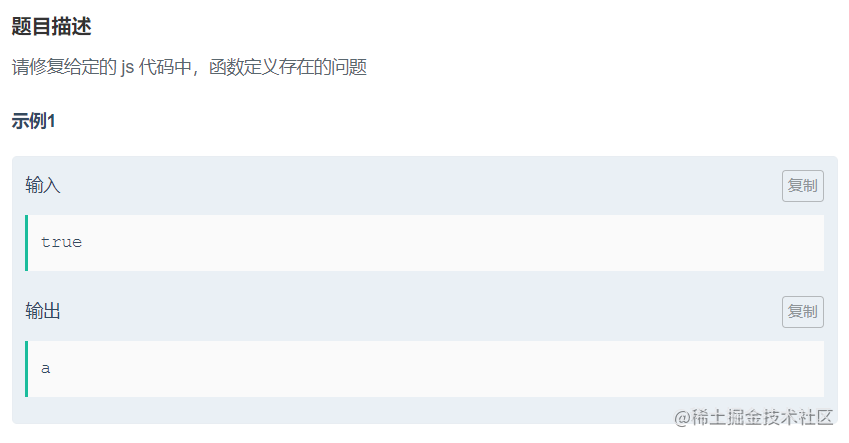原代码：

``````function functions(flag) {
if (flag) {
function getValue() { return 'a'; }
} else {
function getValue() { return 'b'; }
}

return getValue();
}

``````function functions(flag) {
if (flag) {
var getValue = function () { return 'a'; }
} else {
var getValue = function () { return 'b'; }
}

return getValue();
}

## 17、正确的使用 parseInt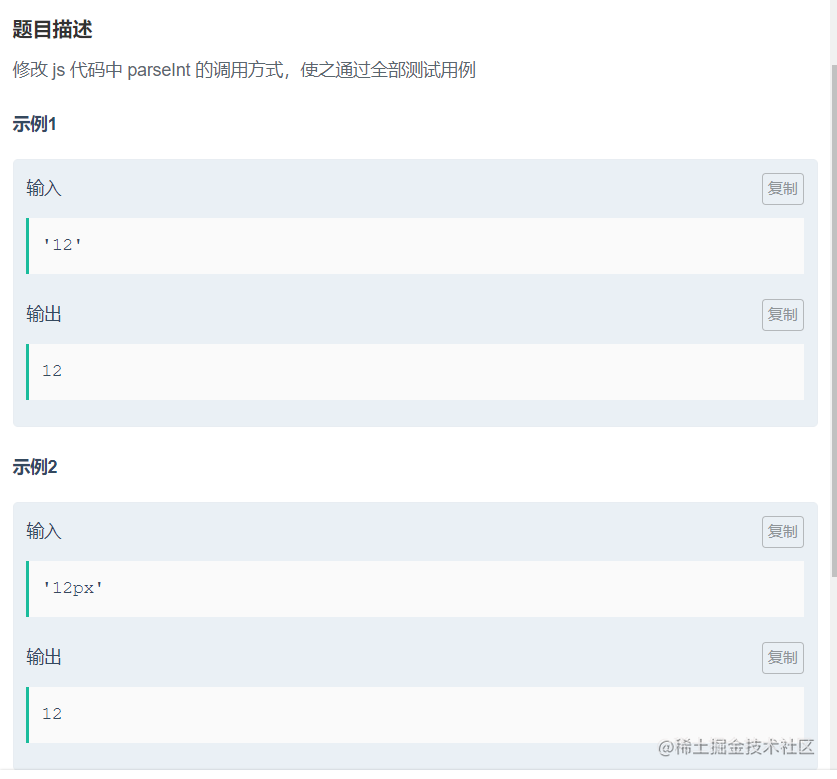原代码：

``````function parse2Int(num) {
return parseInt(num);
}

``````function parse2Int(num) {
return parseInt(num,10);
}

1.只有字符串中的第一个数字会被返回。

2.如果字符串的第一个字符不能被转换为数字，那么 parseFloat() 会返回 NaN。 3.如果参数 radix 小于 2 或者大于 36，则 parseInt() 将返回 NaN。

## 18、完全等同

``````function identity(val1, val2) {
if(val1===val2) {
return true;
}
else{
return false;
}
}

## 19、计时器

``````function count(start, end) {
//立即输出第一个值
console.log(start++);
var timer = setInterval(
function()
{
if(start <= end){
console.log(start++);
}else{
clearInterval(timer);
}
},100);
//返回一个对象
return {
cancel : function()
{
clearInterval(timer);
}
};
}

setInterval(code,millisec) 方法可按照指定的周期（以毫秒计）来调用函数或计算表达式。

code 是要调用的函数或要执行的代码串，millisec 是周期性执行或调用 code 之间的时间间隔，以毫秒计。

setInterval() 方法会不停地调用 code 函数，直到 clearInterval() 被调用或窗口被关闭。由 setInterval() 返回的 ID 值可用作 clearInterval() 方法的参数。 clearInterval() 方法可取消由 setInterval() 设置的 timeout，其中的参数必须是由 setInterval() 返回的 ID 值。

## 20、流程控制

``````function fizzBuzz(num) {
if(num%3==0 && num%5==0){
return 'fizzbuzz';
}
else if(num%3==0){
return 'fizz';
}
else if(num%5==0){
return 'buzz';
}
else if(num==null || typeof num != 'number'){
return false;
}
else{
return num
}
}

## 21、函数传参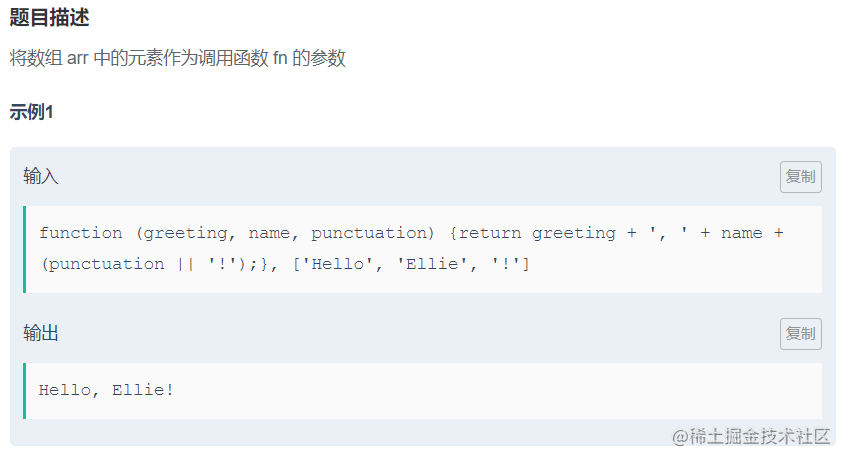方法一：apply/call

``````function argsAsArray(fn, arr) {
return fn(arr,arr,arr);
}

function argsAsArray(fn, arr) {
return fn.apply(fn, arr);
}

//或者
function argsAsArray(fn, arr) {
return fn.apply(this, arr);
}

function argsAsArray(fn, arr) {
return fn.call(fn, arr,arr,arr);
}

//或者
function argsAsArray(fn, arr) {
return fn.call(this, arr,arr,arr);
}

function.apply(newObj[, argsArray]) 方法可以修改指定函数的调用对象。function 是调用对象将被修改的函数，newObj 是函数的新调用对象，argsArray 是传递给function函数的参数，数组或者arguments对象。

apply 方法是将传递给函数的参数放入一个数组中，传入参数数组即可。

function.call(newObj[, arg1[, arg2[, [,…argN]]]]) 方法的作用和 apply() 方法类似，只有一个区别，就是 call() 方法接受的是若干个参数的列表，而 apply() 方法接受的是一个包含多个参数的数组。

``````function argsAsArray(fn, arr) {
return  fn(...arr);
}

## 22、函数的上下文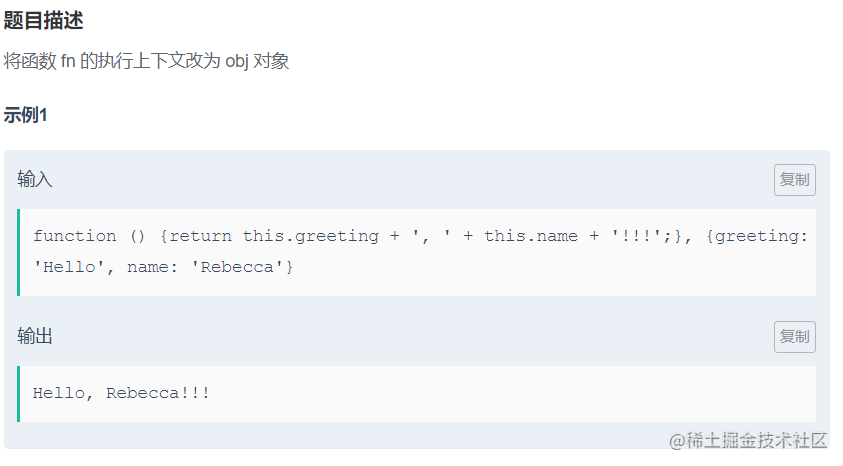这个题目考察的是改变this指向

``````//apply
function speak(fn, obj) {
return fn.apply(obj);
}
//call
function speak(fn, obj) {
return fn.call(obj);
}
//bind
function speak(fn, obj) {
return fn.bind(obj)();
}

## 23、返回函数

``````function functionFunction(str) {
return f = function (obj) {
return str + ', ' + obj;
}
}

## 24、使用闭包

``````function makeClosures(arr, fn) {
let result = [];
for (let i of arr) {
result.push(()=>{
return fn(i)
});
}
return result;
}
// 也可以换成forEach

## 25、二次封装函数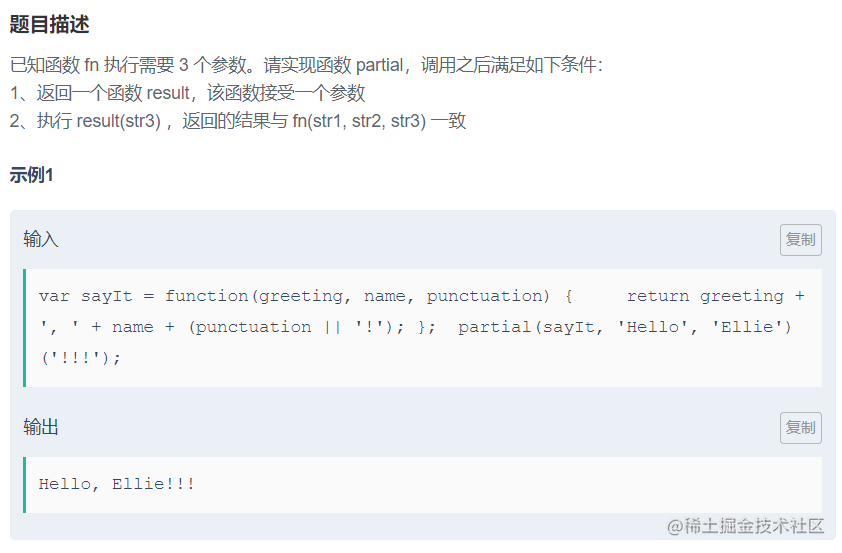因为没有要求函数内部this指向和作用域，所以可以不用 call 和 apply 方法。

``````function partial(fn, str1, str2) {
return result = function (str3) {
return fn(str1, str2, str3)
};
}

## 26、使用 arguments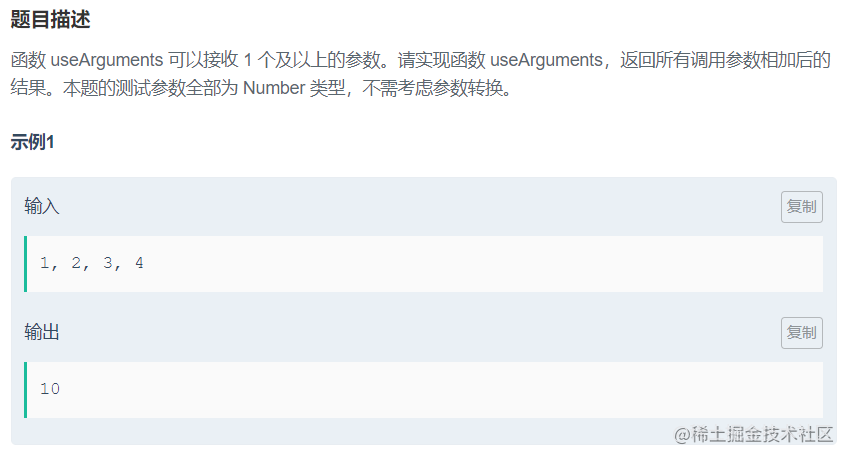方法一：eval

``````function useArguments() {
let arr=Array.prototype.slice.call(arguments)//把arguments类数组转化为数组
return eval(arr.join("+"));//求和
}

``````function useArguments() {
let result = Array.prototype.reduce.call(arguments,function(a,b){return a+b;});
return result;
}

## 27、使用 apply 调用函数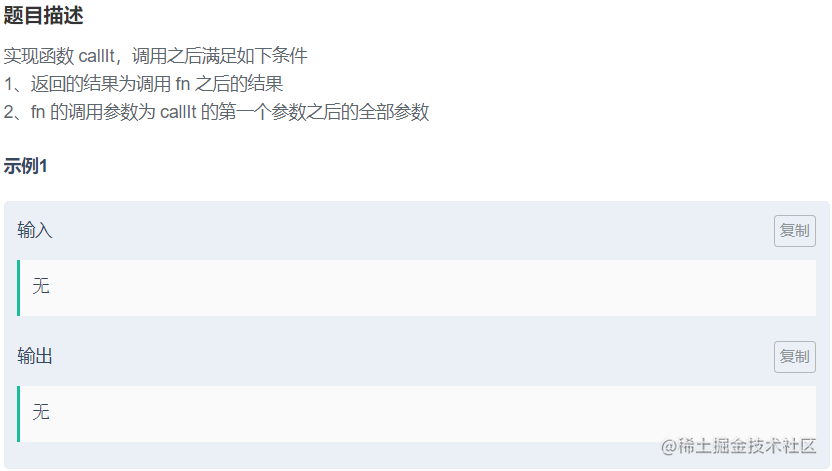21题已经讲过，不再赘述

``````function callIt(fn) {
return fn.apply(this, [].slice.call(arguments, 1))
}

## 28、二次封装函数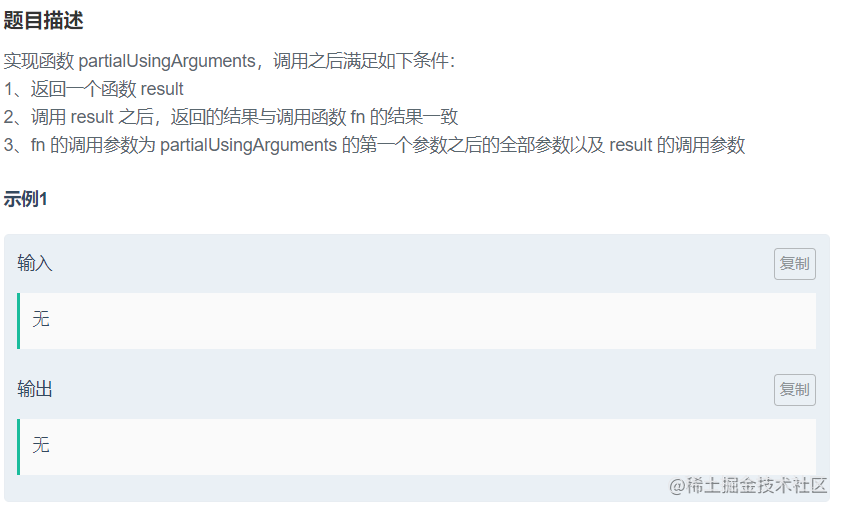注意与25题的要求区别。入门级别，不多说，是个人都会

``````function partialUsingArguments(fn) {
//先获取p函数第一个参数之后的全部参数
var args = [].slice.call(arguments,1);
//声明result函数
return result = function(){
//使用concat合并两个或多个数组中的元素
return fn.apply(this, args.concat( [].slice.call(arguments) ));
};
}

## 29、柯里化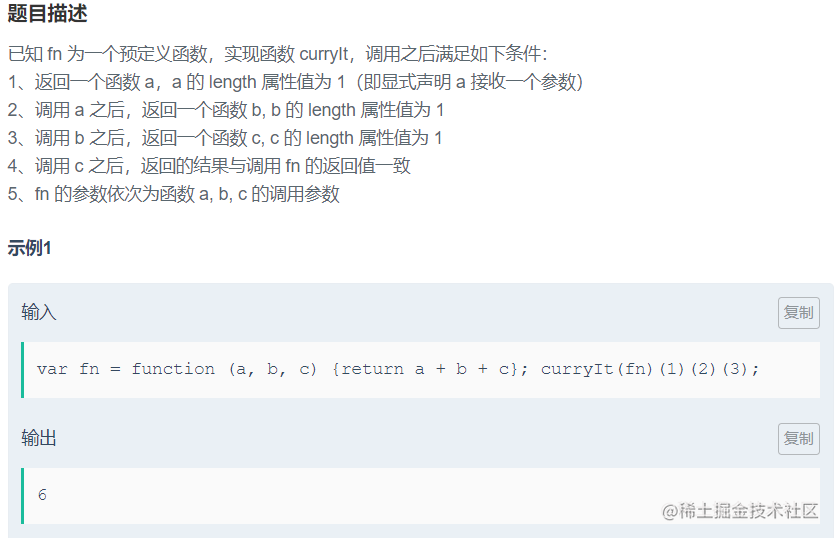柯里化是把接受多个参数的函数变换成接受一个单一参数(最初函数的第一个参数)的函数，并且返回接受余下的参数且返回结果的新函数的技术。

``````function curryIt(fn) {
var args = [];
var result = function(arg){
args.push(arg);
if(args.length < fn.length){
return result;
}else
return fn.apply(this,args);
}
return result;
}

## 30、或运算

``````function or(a, b) {
return (a || b);
}

## 31、且运算

``````function and(a, b) {
return  (a && b);
}

## 32、模块

``````function createModule(str1, str2) {
let obj = {
greeting: str1,
name: str2,
sayIt: function(){
return obj.greeting+", "+obj.name;
}
};
return obj;
}

## 33、二进制转换(十进制转二进制)

``````function valueAtBit(num, bit) {
let res = num.toString(2);
return sre[res.length - bit];
}

``````当调用该方法的对象不是 Number 时抛出 TypeError 异常。

## 34、二进制转换(二进制转十进制)

``````function base10(str) {
return parseInt(str,2);
}

## 35、二进制转换(十进制转8位二进制)

``````function convertToBinary(num) {
let res = num.toString(2);
while(res.length<8){
res = '0'+res;
}
return res;
}

## 36、乘法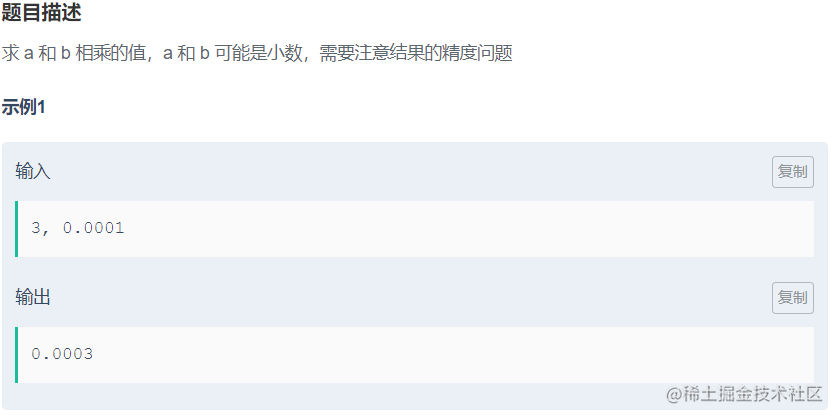本题要注意题目要求，我们需要考虑精度，且保证以小数点后位数多的为准。以下写法是不能通过的

``````// 这种写法是通不过的
function multiply(a, b) {
return a*b;
}

``````function multiply(a, b) {
// 先将数字转换为字符串
let str1 = a.toString();
let str2 = b.toString();
// 获取两个数的小数位数
let lenA = (str1.indexOf('.')==-1)?0:(str1.length-str1.indexOf(".")-1);
let lenB = (str2.indexOf('.')==-1)?0:(str2.length-str2.indexOf(".")-1);
// 比较两数的精度/位数，精度大的作为结果数精度
let len = Math.max(lenA,lenB);
// 运算结果
let result = parseFloat(a*b).toFixed(len);
return result
}

• toFixed(num):`toFixed()` 方法可把 Number 四舍五入为指定小数位数的数字; 参数num: 代表小数位数；
``````let num = 5.56789; num.toFixed(2); ==>5.57

• parseFloat(string): `parseFloat()`函数可解析一个字符串，并返回一个浮点数；参数 string 可为数字可为字符串，当参数为字符串时，判断个字符是否是数字，如果是，则对字符串进行解析，直到到达数字的末端为止，然后以数字返回该数字，如果不是，返回NAN；
``````parseFloat("43ar4s2")；==>43
parseFloat("qwar4s2")；==>NAN

## 37、改变上下文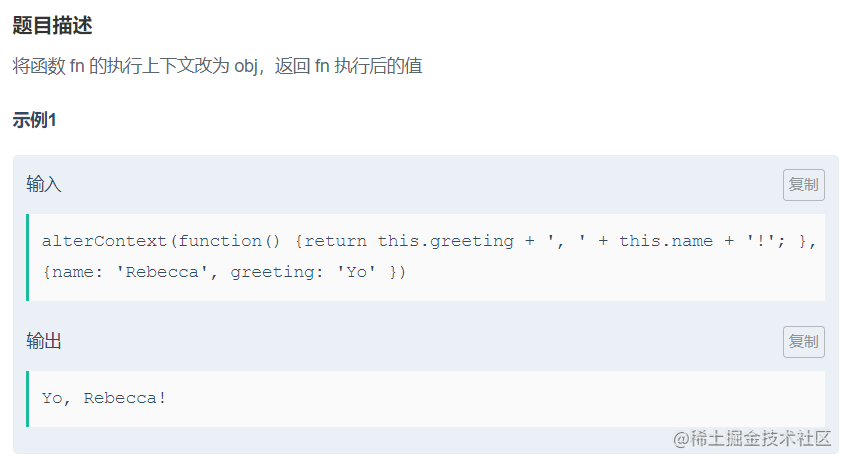前面有讲过，不再赘述

``````function alterContext(fn, obj) {
//return fn.call(obj);
//return fn.apply(obj);
return fn.bind(obj)();
}

## 38、批量改变对象的属性

``````function alterObjects(constructor, greeting) {
constructor.prototype.greeting = greeting;
}

prototype 属性可以向对象添加属性和方法。 这是原型链的知识：当查找一个对象的方法或者是属性时，首先会在该对象中寻找，如果找到则返回如果实例对象自身不存在该属性，则沿着原型链往上一级查找，找到时则输出，不存在时，则继续沿着原型链往上一级查找，直至最顶级的原型对象Object.prototype，如还是没找到，则返回undefined

## 39、属性遍历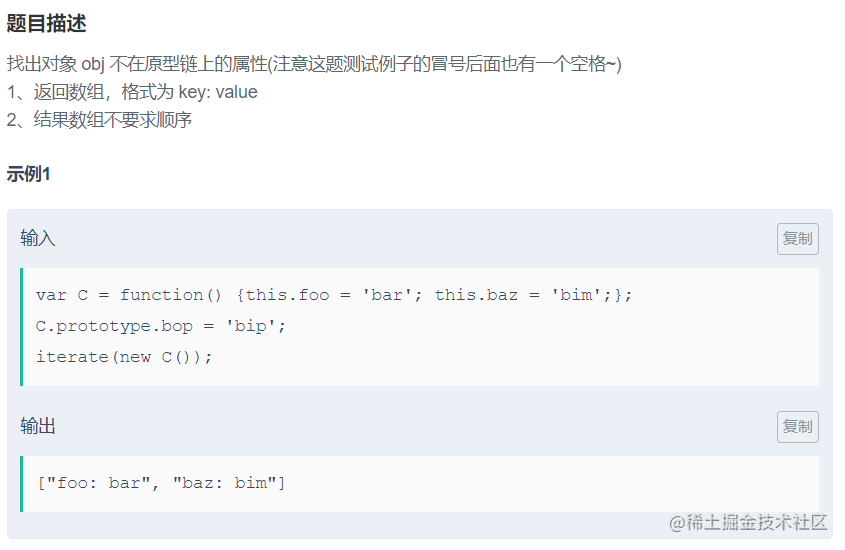方法很多凡是有遍历功能的方法都可以

``````//运行时间：1148ms 占用内存：77864k
function iterate(obj) {
let result = [];
for(let key in obj){
if(obj.hasOwnProperty(key)){
result.push(key+': '+obj[key]);//使用obj.key部分代码不能通过
}
}
return result;
}

``````//运行时间：1133ms 占用内存：77828k
function iterate(obj) {
return Object.getOwnPropertyNames(obj).map(key=>{
return key+": "+obj[key];
});
}

`Object.getOwnPropertyNames()`方法返回一个由指定对象的所有自身属性的属性名（包括不可枚举属性但不包括Symbol值作为名称的属性）组成的数组。 方法三：forEach

``````//运行时间：1173m  占用内存：77872k
function iterate(obj) {
let arr=Object.keys(obj);
let resArr=[];
arr.forEach(item=>{
resArr.push(item+': '+obj[item])
})

return resArr

}

## 40、判断是否包含数字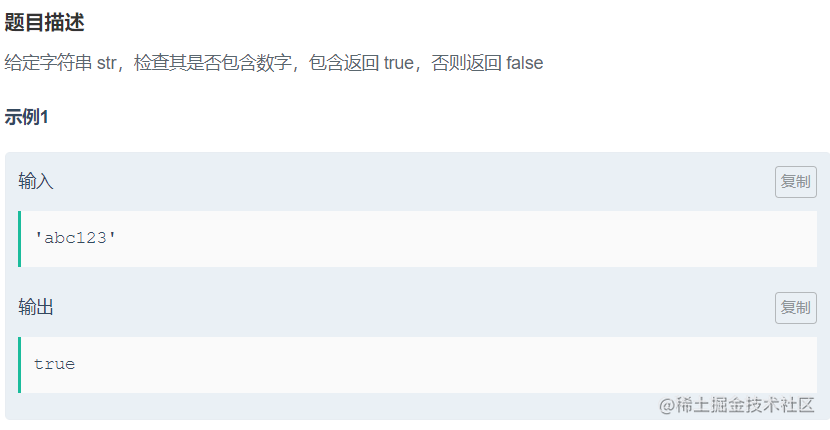方法一：正则test/match

``````function containsNumber(str) {
// return /\d/.test(str);
return !!str.match(/\d/g);
}

``````function containsNumber(str) {
for(let i=0;i<10;i++){
if(str.indexOf(i)!=-1){
return true;
}
}
return false;
}

``````function containsNumber(str) {
let res = str.split('')
for(let i = 0;i<res.length; i++){
let temp = res[i].charCodeAt();
if(temp>=48 &&temp<=57){
return true
}
}
return false
}

``````function containsNumber(str) {
let res = str.split('')
for(let i = 0;i<res.length; i++){
let temp = Number(res[i]);
if(temp){
return true
}
}
return false
}

## 41、检查重复字符串

``````function containsRepeatingLetter(str) {
return /([a-zA-Z])\1/.test(str);
}

``````function containsRepeatingLetter(str) {
let reg = /[a-zA-Z]/;
for(let i = 0;i < str.length;i++){
if(str.charAt(i)==str.charAt(i+1)&&reg.test(str[i])){
return true;
}
}
return false;
}

## 42、判断是否以元音字母结尾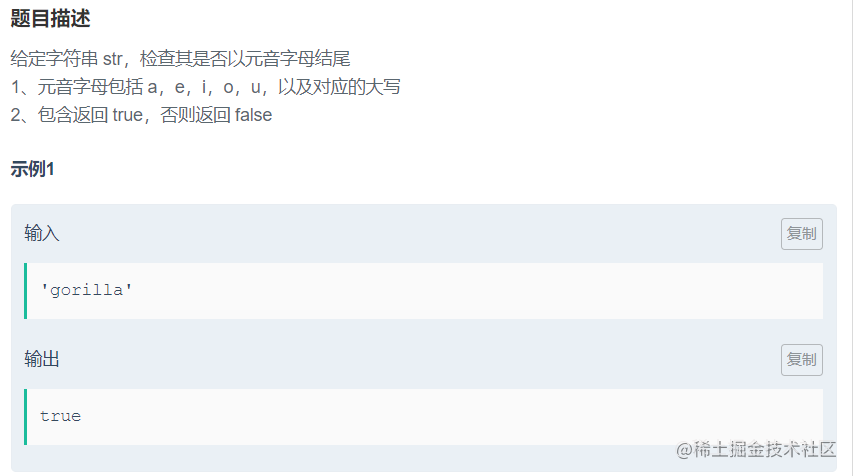首先确定元音集合`[a,e,i,o,u]`，然后是以元音结尾，加上 \$ ，最后通配大小写，加上i。

``````function endsWithVowel(str) {
return /[a,e,i,o,u]\$/i.test(str);
//或者
// return /(a|o|e|i|u)\$/gi.test(str);
}

``````function endsWithVowel(str) {
return str && ("aeiouAEIOU".indexOf(str[str.length-1]) > -1);
}

## 43、获取指定字符串

``````function captureThreeNumbers(str) {
//声明一个数组保存匹配的字符串结果
let arr = str.match(/\d{3}/);
//如果arr存在目标结果，则返回第一个元素，即最早出现的目标结果
if(arr){
return arr;
} else{
return false;
}
}

## 44、判断是否符合指定格式

``````function matchesPattern(str) {
return/^\d{3}-\d{3}-\d{4}\$/.test(str);
}

``````本题需要注意格式，开头 ^ 和结尾 \$ 必须加上来限定字符串，3个数可表示为\d{3}，4个数则为\d{4}，{n}表示前面内容出现的次数。正则表达式可写作 /^ \d{3}-\d{3}-\d{4}\$ / ，有相同部分\d{3}-，因此也可写作 /^(\d{3}-){2}\d{4}\$/ 。

## 45、判断是否符合 USD 格式

``````function isUSD(str) {
return /^\\$\d{1,3}(,\d{3})*(\.\d{2})?\$/.test(str);
}

``````? 匹配前面一个表达式0次或者1次。等价于 {0,1}。

(注意第二个 ? 前面的匹配表达式是 e 而不是 le) 以及 'oslo' 中的'l'。

* 匹配前一个表达式0次或多次。等价于 {0,}。

. (小数点)匹配  除了换行符（\n）之外的任何单个字符。

## 💕看完三件事:

1. 点赞 | 你可以`点击——>收藏——>退出`一气呵成，但别忘了点赞🤭
2. 关注 | 点个关注，下次不迷路😘
3. 也可以到GitHub拿我所有文章源文件🤗